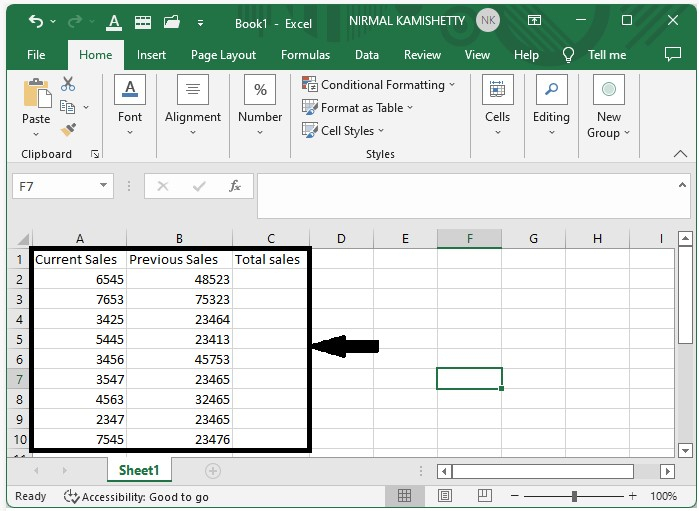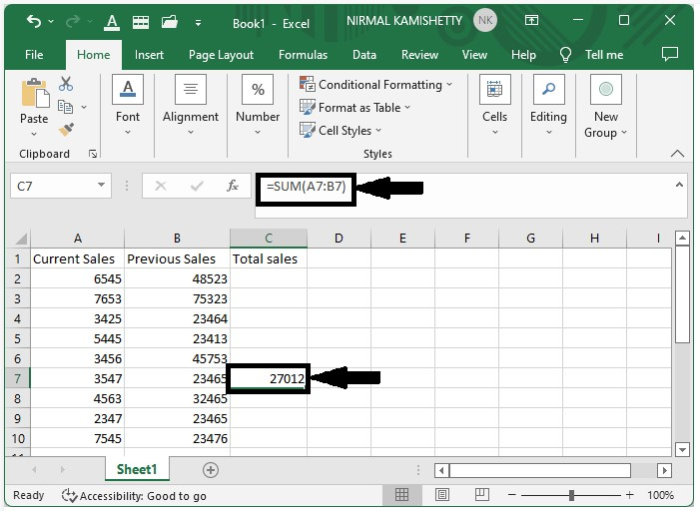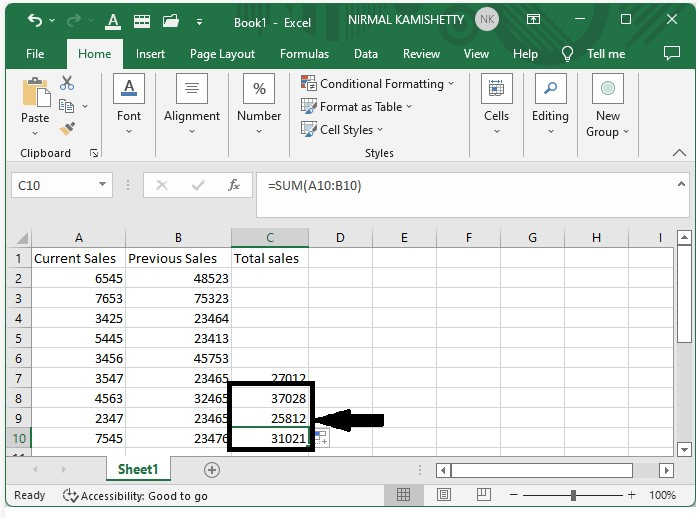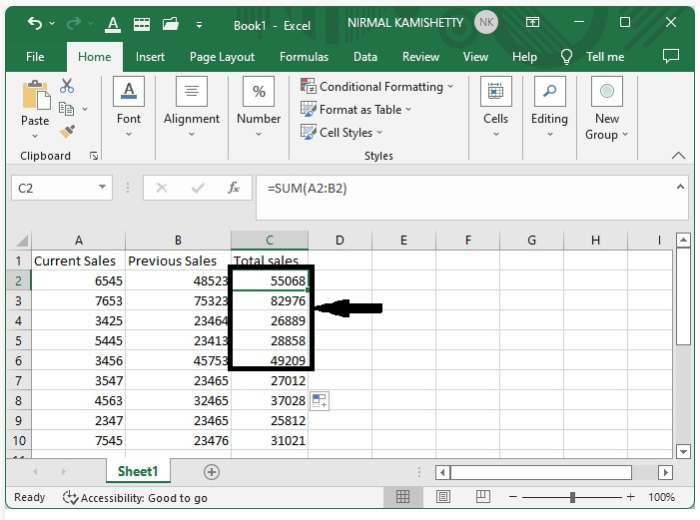# How to Apply Same Formula to Multiple Cells in Excel?

We have tried to apply the same formula to multiple cells in Excel many times. This is the most commonly used process in Excel to get the outputs for multiple cells at once. In Excel, there are several methods for applying the same formula to multiple cells; one method is to select multiple cells while using the formula for formatting and data validation. Then the other way of applying the same formula to multiple cells is to drag the result. Then drag in any direction: up, down, left, right, or any combination of these.

Read this tutorial to understand how you can apply the same formula to multiple cells in Excel.

## Applying the Same Formula to Multiple Cells By Dragging

Here, we will first use a simple formula, then use the auto-fill handle to spread the formula. Let's go over a simple procedure for applying the same formula to multiple cells in Excel by dragging. We will be adding two columns to the example.

Step 1

Let us consider an Excel sheet where the data present is similar to the data shown in the below image.In our case, we can click on cell C7 and enter the formula =SUM(A7:B7) in the formula box, then press Enter to get the result for cell C7, as shown in the image below.Step 2

Drag down from cell C7 until all the results are filled in, as shown in the image below, to get the results for the cells below C7.Step 3

Now, to get the results for the cells above C7, pull up from the cell C7 until all the results are filled in as shown in the below image.## Conclusion

In this tutorial, we used a simple example to demonstrate how you can apply the same formula across multiple cells in Excel to highlight a particular set of data.